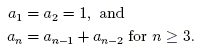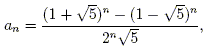The term of the Fibonacci sequence is .

The Fibonacci sequence is the sequence of integers 1, 1, 2, 3, 5, 8, 13, 21, 34, 55, 89, 144, 233, 377, 610, 987,... It is defined byFor example, 1 + 1 = 2, 1 + 2 = 3, 2 + 3 = 5, 3 + 5 = 8, etc.

The above calculator computes the nth term of the Fibonacci sequence for n ≤ 1474 and will subsequently compute the previous and next terms. It uses Binet's formula,which is an explicit formula for the nth term of the Fibonacci sequence.